## Wednesday, November 18, 2015

### Buoys to Maui travel times and Maui's shadow lines

TRAVEL TIMES

NW buoy to Maui

I found the time to do some investigation on the wave speed topic I mentioned yesterday and found this article that seemed to be the most reliable. I got to it following some links on this other one, which is a really good read if you have time and want to learn more about waves.

From it, I'm gonna quote this sentence: "It's matter of experience and choice as to what size, period, and swell direction each of us prefers, but if you know what the swell conditions are before you set foot in the water, you'll be a lot better prepared."
That is pretty much what this blog is all about.

Let me also point out this page with the world wide great circles, from where you can get the Hawaii great circles for the north pacific and the Hawaii great circles for the south pacific. The great circles allow you to see from what direction a swell from a fetch in a certain position will come and they take into account the curvature of the Earth, which instead gets distorted in the two dimensional maps that we commonly use.

Below is a table that has the following columns:

- P is the period
- S is the speed at which swells travel  in knots (nmph)
- T is the time to cover the 383 nm that separate the NW101 buoy from Maui
- GP's is the approximation of that time if you use the new and improved GP's rule of thumb, which you can easily remember because it goes: 16h at 16s (+1h -1s and viceversa for different periods)

Everything in between (actually everything between 12 and 21 seconds), you can just interpolate by adding and subtracting 1h to the time and 1s to the period. The maximum approximation you get in the 12 to 21s range is 0.7 hours (42 minutes), which is plenty precise for me, seen the non precise nature of these things.

P               S                T               GP's
```10s  =  15.6   =  24.5  =  22 n/a
11s  =  17.16  =  22.3  =  21 n/a
12s  =  18.72  =  20.5  =  20
13s  =  20.29  =  18.9  =  19
14s  =  21.84  =  17.5  =  18
15s  =  23.4   =  16.4  =  17
16s  =  24.96  =  15.3  =  16
17s  =  26.52  =  14.4  =  15
18s  =  28.08  =  13.6  =  14
19s  =  29.64  =  12.9  =  13
20s  =  31.2   =  12.3  =  12
21s  =  32.76  =  11.7  =  11
22s  =  34.32  =  11.2  =  10 n/a
23s  =  35.88  =  10.7  =   9 n/a
24s  =  37.44  =  10.2  =   8 n/a
25s  =  39     =   9.8  =   7 n/a```

Those travelling time are most correct for a swell that comes from 303 degrees, which is where the NW101 buoy sits compared to us. Any difference in the direction will introduce a difference in the travelling time, which for our purposes I would tend to consider negligible. Just remember that both for more northerly and more westerly swells, the time will be less than what the table says, but the energy hitting the buoy will not be exactly what we receive, so it's not worth to put too much effort into calculating the exact arrival time.

-------------------------------------------------------------

North buoy to Maui

The N buoy is 212 nm out at a 42 degrees angle. Here's the travel times for a NE swell coming from exactly 42 degrees:

P               S                T               GP's
```10s  =  15.6   =  13.5  =  13
11s  =  17.16  =  12.3  =  12
12s  =  18.72  =  11.3  =  11
13s  =  20.29  =  10.5  =  10
14s  =  21.84  =   9.7  =  -
15s  =  23.4   =   9.0  =  -
16s  =  24.96  =   8.5  =  8
17s  =  26.52  =   8.0  =  -
18s  =  28.08  =   7.5  =  -
19s  =  29.64  =   7.1  =  -
20s  =  31.2   =   6.8  =  -
21s  =  32.76  =   6.5  =  -
22s  =  34.32  =   6.2  =  -
23s  =  35.88  =   5.9  =  -
24s  =  37.44  =   5.7  =  -
25s  =  39     =   5.4  =  -```

Legenda:
- P is the period
- S is the speed at which swells travel  in knots (nmph)
- T is the time to cover the 212 nm that separate the N buoy from Maui
- GP's is the approximation of that time to provide a rule of thumb that is easy to remember. In this case it could be 10h at 13s and viceversa (with valid interpolation in between) and then you have to remember that 16s will take half of that numbers in hours (8). Most swells coming from the NE don't have elevated periods anyway.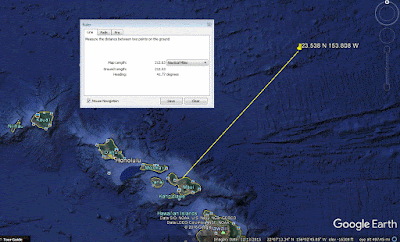Every other direction, either more north or more east will take less time to get here. BUT, it will not be exactly the same energy that's hitting the swell though (that should be more clear in the next picture below). Here's the travel time for a straight north swell, for example.

20sec--30kts--5 h

17sec--26kts--5.8 hrs

14sec--21kts--7.2 hrs

11sec--17kts--9 hrs

Let's take a swell coming from 12 degrees and at 14s, for example. The travel time is 7.6 hours. BUT, as I was saying, that energy that is hitting the N buoy will miss Maui to the east. 7.6 hours later, we're gonna get the energy that would hit a buoy sitting where I put the question mark on the map. Is that energy going to be exactly what is at the N buoy at the same time? Of course not and there's no way to find out the difference which, seen the relatively close distance between the two points, is not going to be too big anyway. Once again, don't get too fixated on the numbers, you see how many approximations there are?-------------------------------------------------------------

Waimea buoy to Maui

The distance is roughly 102 nm and the table below gives you an idea of the travel time.

P               S                T
```10s  =  15.6   =   6.5
11s  =  17.16  =   5.9
12s  =  18.72  =   5.4
13s  =  20.29  =   5
14s  =  21.84  =   4.6
15s  =  23.4   =   4.4
16s  =  24.96  =   4
17s  =  26.52  =   3.8
18s  =  28.08  =   3.6
19s  =  29.64  =   3.4
20s  =  31.2   =   3.2
21s  =  32.76  =   3.1
22s  =  34.32  =   2.9```---------------------------------------------------

Hanalei buoy to Maui

The distance is roughly 195 nm and the table below gives you an idea of the travel time.

P               S                T
```10s  =  15.6   =  12.5
11s  =  17.16  =  11
12s  =  18.72  =  10.4
13s  =  20.29  =   9.6
14s  =  21.84  =   8.9
15s  =  23.4   =   8.3
16s  =  24.96  =   7.8
17s  =  26.52  =   7.3
18s  =  28.08  =   7
19s  =  29.64  =   6.5
20s  =  31.2   =   6.2
21s  =  32.76  =   6
22s  =  34.32  =   5.7```-----------------------------------------------

Samoa buoy to Maui

The Samoa buoy (S on the map, F stands for Fiji instead) is located at 14.265 S 170.494 W which is at an angle of 202 degrees SSW of Maui and at a distance of 2,222 nm. Below are the travelling times of swells coming from that direction.

P               S                  T (days)
```11s  =  17.16  =   5.5
12s  =  18.72  =   5
13s  =  20.29  =   4.5
14s  =  21.84  =   4.2
15s  =  23.4   =   4
16s  =  24.96  =   3.7
17s  =  26.52  =   3.5
18s  =  28.08  =   3.3
19s  =  29.64  =   3.1
20s  =  31.2   =   3d
```

As you can see, there's a narrow path between the New Zealand's north island and Fiji that is unobstructed. The direction of that path is 210. The biggest south swells for Hawaii, usually come from just east of New Zealand, an area which is at 200 south of us.-----------------------------------------------

Fiji to Maui

If it happens that you know when a swell hits Fiji, here's the travel times from there to Hawaii.

P               S                  T (days)
```11s  =  17.16  =   6.8
12s  =  18.72  =   6.2
13s  =  20.29  =   5.7
14s  =  21.84  =   5.3
15s  =  23.4   =   5
16s  =  24.96  =   4.7
17s  =  26.52  =   4.4
18s  =  28.08  =   4.1
19s  =  29.64  =   3.9
20s  =  31.2   =   3.7```-----------------------------------------------

East of New Zealand to Maui

Travel time from east of New Zealand, where most of the south swells form.

P               S                  T (days)
```11s  =  17.16  =   10.7
12s  =  18.72  =   9.8
13s  =  20.29  =   9
14s  =  21.84  =   8.4
15s  =  23.4   =   7.8
16s  =  24.96  =   7.3
17s  =  26.52  =   6.9
18s  =  28.08  =   6.5
19s  =  29.64  =   6.2
20s  =  31.2   =   5.9```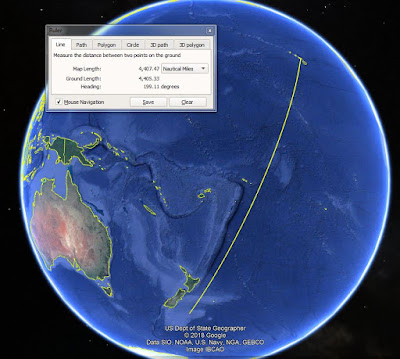-------------------------------------------------------------------------------

Hookipa on NW swells
Below you have a line from Hookipa to the tip of Molokai and the angle says 305.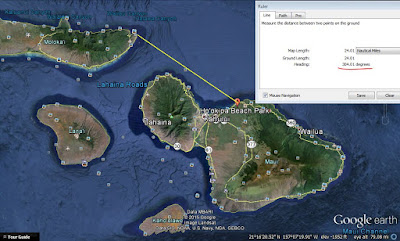Pauwela
The Pauwela buoy is 6 miles offshore of Hookipa.
As you can see, the Molokai shadow for the buoy is 299. Hookipa's is 305. It's not a huge difference, but it is a difference. That means that every time there is a swell that is more west than 300, the buoy will show more energy than the one that will eventually hit Hookipa. Let alone the rest of the coastline towards Kahului.

Peahi
Peahi's line instead sits at 298, so what the buoy reads is what is going to get there. Quite convenient, I'd say.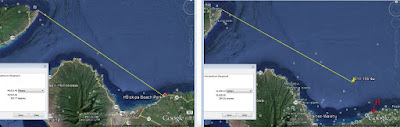Hookipa on East swells
The map below shows that actually the orientation of Maui's north shore from Hookipa to Kahului is roughly 65 degrees.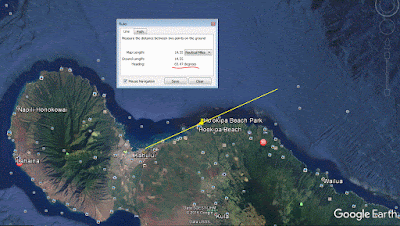This second one though, shows that Hookipa is a bit more exposed with a geometrical shadow line placed at 68 degrees. Don't forget that the higher the period, the more those geometrical shadow lines between emerged land points lose their significance, because a long period swell will be effected more by the submerged reefs, refract around them and eventually end up in places you would not suspect just by looking at a map. They do work much better for shorter periods though (like today's windswell, for example), since these are less sensitive to the bottoms.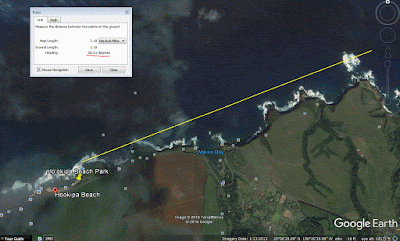A very good example of bottom influenced behavior is the Waiehu coast.
The picture below shows a shadow line from the Mokeehia Island to the end of the Kahului harbor west pier of 332. But you can surf on that side with swells that are more west than that, as long as they're long period and big enough.This explains even better what submerged reefs can do to swells. Let's imagine there's a big NW swell from a direction more west than what I just said. The swell will refract on Kahakuloa and let's imagine it arrives to the Waiehu golf course area with a direction of 320 (yellow line). In front of the golf course there's a reef (R) that sticks out quite a bit.

The swell will refract on this reef too and bend enough to hit the spots in the area that I circled with the letter W (waves!) and completely miss the area that I circled with F (flat). The funny thing is that can happen even with a swell coming from 335. You think it's gonna get to the F area because it's unblocked by emerged lands, but it will miss it anyway because the reef R will "suck" most of the set (which will be big there) and leave the F area flat. That happens a lot in all surf spots, it's just that most surfers are not aware of this. When the WSL commentators do a proper "break breakdown" for Pipeline or Waimea, for example, these concepts appear also very clear.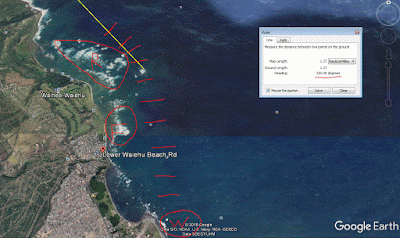Waiehu on East swells.
Here's the shadow line for Waiehu: 80 degrees. Which means that a good part of a 90 degrees easterly energy will be blocked there too, but for sure there will be more than at Hookipa. Relatively short periods like 7-9s (typical windswell) still wrap around land points, but can't do as sharp as a turn as long period ones. Hookipa has a shadow line of 65 degrees and it requires a much sharper bend, which justifies the sentence above.Hanamanu.
The direct hits for the inside left are between 0 and 50 degrees, but, if the swell is big enough, it works well outside that. The right prefers a bit of west in the swell.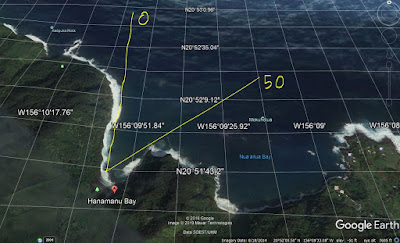Honolua
Below is the shadow line for Honolua that sits at 335 degrees. Anything more west than that, will have to bend around Molokai to hit the bay. The bigger the size and the higher the period, the more that will happen.
I also drew some lines representing a swell coming from 360 instead to show how it will travel unrefracted till the bay and then, if it's big enough, start bending around the coast line. If a straight north swell is big enough, the wrap will be felt all the way down to Puamana. Same can happen for swells coming from the NE quadrant, as long as they are big/long period enough.

And if a swell from 335 or even more west than that is big/long period enough, you can imagine it doing an S turn: first refracting on Molokai and changing its direction to more straight north and then refracting on west Maui and changing its direction to more west again. All this, of course, happens to the expense of the energy of the swell, but it's pretty amazing that waves can do that.

Kanaha
This is the shadowing angle for a very popular break: Kanaha. The angle there seems to be 319 and is due to the West Maui Mountain more than Molokai. So, a swell coming from 325 for example, shouldn't feel any influence from either one.
A swell coming from 320 will feel some influence from the WMM instead.
I've watched for years big breakers off the golf course in Waiehu in such occasions, because the reef bends the sets towards itself leaving Sand Piles pretty much flat. All the next breaks down the coast towards Kahului instead will have plenty waves.
Anything more west than that, will still eventually get to Kanaha (and the Waiehu coast) depending on size and period. You can imagine Molokai doing a first part of the refraction for the swells between 310 and 320 and Kauai and Oahu doing adding more refraction from swells more west than that. In other words, good luck at trying to predict the size of the waves at Big Left on a 10f 15s swell from 305. That's when you just get in the car and go check. For sure you can expect less consistency than if it was hitting straight without refraction.Kihei
Let's talk also about the Kihei coast and the westerly swells.
Google Earth shows the shadow line from Lanai to Kalama park in Kihei as 273 degrees. Anything from there to straight west, doesn't get blocked/refracted and will have a more direct impact.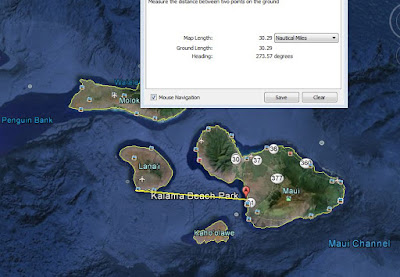Ahihi
A little better angle applies to Ahihi Bay: 283.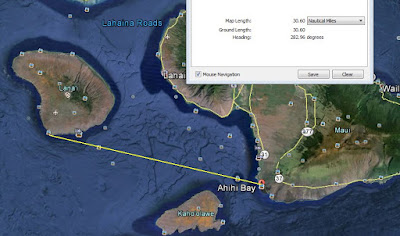But don't forget that a the bigger the size and the period of a swell, the more the waves have the ability to refract around land points. The photo below shows that as long as the swell is 290 or more west, the south point of Lanai will refract energy that has not been refracted by Ni'iahu. But if a swell is big and long period enough, even if it comes from directions more north of 290, it could still refract first over the south point of Ni'iahu, change its direction into 290 or more west, and then refract again over Lanai and hit Maui. I remember one coming from around 300 that provided double overhead waves to Kihei.

It all depends on each single swell and there's no mathematical/geometrical rules you can apply that work all the times.
I've see too many times very similar swells doing very different things.
That is also because if the swell has a direction at the NW buoy, that doesn't mean that the swell hitting the south point of Ni'iahu will have the exact same direction. It is possible that it will be more west there and that the waves will be bigger than you would expect based on these information I'm providing.
So this is just a reference to try to guess when it's worth to get in the car (or check the webcams).Another possible (but rare) possibility for the energy of a WNW swell to reach Lahaina and Kihei is to squeeze in the channel between Lanai and Molokai. The picture below shows the shadow line from Ni'iahu, which is around 285.The close up below shows a possible refraction pattern that such swell might have.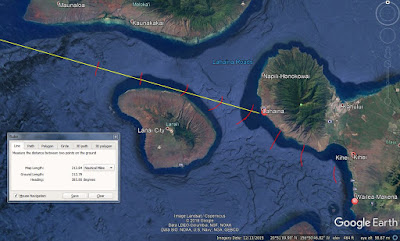La Perouse
And for the very rare ESE pulses, here's the shadowing angle from the Big Island to La Perouse: 161. I like even numbers, so I'm gonna try to remember 160.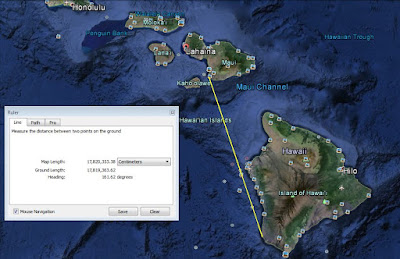Maalaea and Lahaina on South swells
As you can see, the tight opening that Maalaea has to the east of Kahoolawe is only between 165 and 185. And you can't even consider all of those 20 degrees "unaffected" by the uninhabited island. Directions close to the edges will still be refracted and diminished by it. Best direction would be 175.

Lahaina has a much better "view", as its angle goes from 185 to 232. As a consequence, it likes a bit of west in the south swells.Ukumehame on South swells
The shadow is between 170 and 200 degrees. Nonetheless, I've seen it pumping on straight south swells.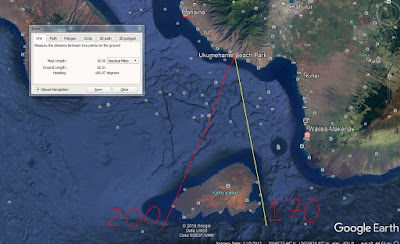Ukumehame and Lahaina on West swells
The map below shows that any westerly swell that comes from more north than 286 will be blocked by Ni'iahu. Once a WNW swell makes it to the channel between Molokai and Lanai, the range to hit Lahaina is 283 to 290. But straight lines are not a correct representation of reality. Waves refract around not only emerged lands, but also submerged ones too, as long as they are shallow enough (the longer the period, the deeper the waves will feel the bottom), so those angles I calculated are just an indication.

If they're more west than 286, as long as they're big/long period enough, some swells might also hit the Lahaina side by either wrapping around the north or the south tip of Lanai or both. Below is an illustration of what I think a straight west swell would do.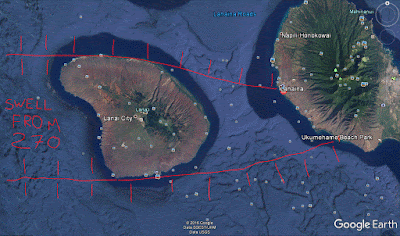And these are the angles the swell needs to have to hit directly (or the ones it will have after the refraction over Lanai).Southern tip of Maui and Big Island shadow on ESE swells.
The photo below shows a shadow line of 118 degrees.Whatever E to ESE energy makes it in the channel between Maui and Big Island will have to wrap further to make it to the road accessible surf spots. For example, Carter's has a shadow line of 158 degrees.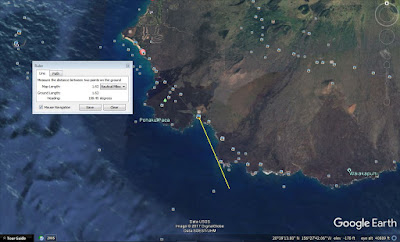Ukumehame and Big Island shadow on SSE swells.

The line below is at 160 degrees. Lahaina instead has a shadow line at 158 degrees. So that's a very narrow corridor that swells coming from around 160 degrees have to get into. That's why I always assume that Big Island together with Kahoolawe block pretty much everything that comes from around 160. But if it's big enough, it might squeeze in there. Anything between 160 and 120 should be completely blocked by the Big Island.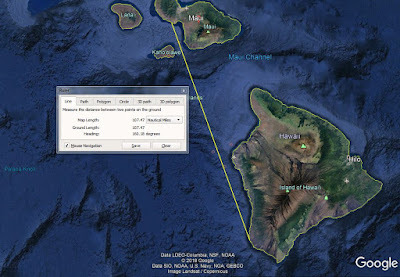Australia and New Zealand's remote shadow lines.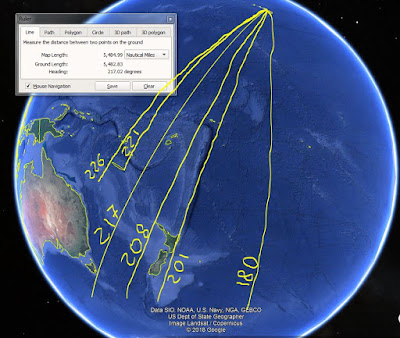------------------------------------------------------------------------------

Lastly, let's first have a quick look at Oahu.

Below is the straight line that connect the NE tip of Kauai with Pipeline. The direction is around 295. For Haleiwa it's around 298. You might think that anything less west than 295 doesn't get blocked at all, but it's not that black and white. A swell from 300 will still refract and bend on all the upstream islands and the result of that in terms of energy loss and direction after the refraction is really hard to quantify.

Here's the article on surfline that explains a bit of that and the travelling time. For convenience, I'll copy it here.

51001 buoy is 170 nm WNW from Hanalei Bay, Kauai and 255nm NW ~300 from Waimea Bay, Oahu.

For a NW swell around 300degrees on the 51001 buoy...

Wave period--Wave speed--Arrival time on North Shore

20sec--30kts--8.3 hrs

17sec--26kts--9.5 hrs

14sec--21kts--12 hrs

11sec--17kts--15 hrs

A more Northerly swell would arrive a little sooner on the North Shore and a more Westerly swell a little later. However, a WNW swell between 270-295 degrees has to contend with the Kauai shadow and will result in 50-70% less energy getting to the North Shore.

Hope this helps some, aloha,

Jonathan Warren
Surfline Forecaster/Meteorologist

First let me point out a little mistake that I believe is in there. If the NW buoy is at 300 degrees from Waimea, that's the direction of a swell that will take the longest time from the buoy to Waimea. Anything more north will take less time, but also anything from more west will take less time. It's a simple geometrical concept. But that's a very unimportant detail, since there's not that many swells from less than 300 and when there are (like today), there's more important things that happen to them, like refraction.

The article says "if it comes from 270-295, the Kauai shadow will result in 50-70% less energy.
But at the same time, long period energy refracts and bends around land points much more than short period ones, so there really isn't a magic formula for that, IMO.
It's more your local knowledge and observations that will have to guide you.